Build Math Skills with a Fun and Challenging App!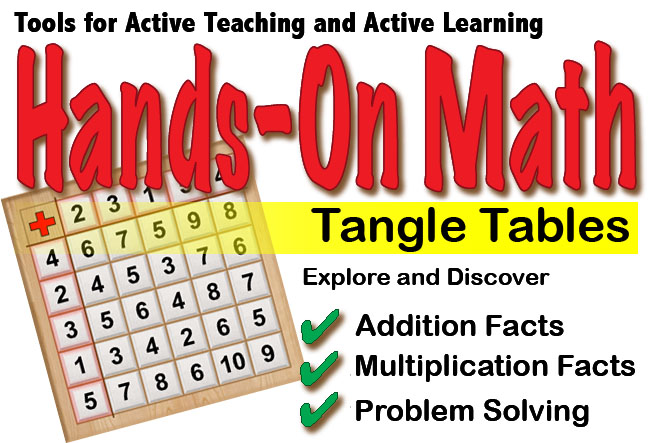Hands-On Math Tangle Tables provides a fun and challenging way to practice addition and multiplication skills, factoring, finding common divisors and problem solving. It is a perfect classroom tool for core mathematics instruction and enrichment. While using this app students will use mathematical thinking skills to solve randomly generated Tangle Tables. Students will find the puzzles as intriguing as Sudoku, but also relevant to their mathematics curriculum. Encourage your students to try Tangle Tables, and as they do you will see them get better at many basic math skills. Tangle Tables offers 12 levels of difficulty. Younger learners will benefit from the settings where the challenge is to find the sum or product and complete the table. For older students teachers may want to use some of the options where the challenge is to use problem solving skills to determine the appropriate addends or multipliers for a randomly generated Tangle Tables. Levels: Level 1: Addition Skills - Whole Numbers 1-5, Find the Sums Level 2: Addition Skills - Whole Numbers 1-10, Find the Sums Level 3: Addition Skills - Whole Numbers 1-5, Find the Missing Addends and Sums Level 4: Addition Skills - Whole Numbers 1-10, Find the Missing Addends and Sums Level 5: Addition Skills - Whole Numbers 6-10, Find the Sums Level 6: Addition Skills - Whole Numbers 6-10, Find the Missing Addends and Sums Level 7: Multiplication Skills - Whole Numbers 1-5, Find the Products Level 8: Multiplication Skills - Whole Numbers 1-10, Find the Products Level 9: Multiplication Skills - Whole Numbers 1-5, Find the Missing Factors and Products Level 10: Multiplication Skills - Whole Numbers 1-10, Find the Missing Factors and Products Level 11: Multiplication Skills - Whole Numbers 6-10, Find the Products Level 12: Multiplication Skills - Whole Numbers 1-10, Find the Missing Factors and Products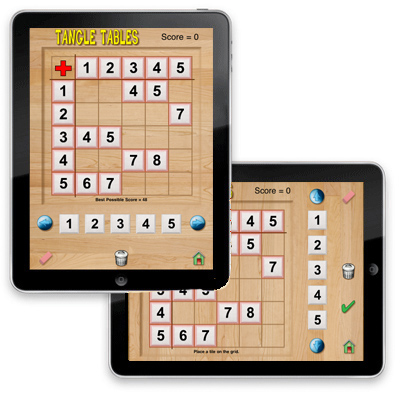Problem Solving and Skill Building Learning addition and multiplication facts can be fun. Tangle Tables presents addition and multiplication practice activities in a logical way. At the beginning levels the addends or multipliers are arranged in order so as the students solve the puzzle a number pattern emerges. Math makes sense! Hands-On Math Tangle Tables creates a virtual mathematical playground right on your iPad. It simulates the use of a popular math manipulative (Number Tiles) and combines it with a creative problem solving activity (Tangle Tables). It is perfect for elementary and middle school classrooms. Enrich your mathematics curriculum with Hands-On Math Tangle Tables. Visualization is one of the best ways to help students explore and discover mathematics. Students who use Tangle Tables will see patterns related to addition and multiplication facts. In addition students will use mathematical reasoning to solve each Tangle Tables. Using Hands-On Math Tangle Tables students can develop the following important mathematical concepts: Addition Facts Multiplication Facts Factoring Logic and Problem Solving Mathematical ReasoningWe recommend that you open the Instructor's Guide PDF from your iPad in a new browser page and save the document in your iBooks app. Addition Tables Multiplication Tables Problem Solving Features: Interactive Tangle Tables for Interaction and Discovery. Supports Common Core Objectives Focuses on Problem Solving Strategies. Problem Solving Activities which Can Be Solved Using Virtual Manipulatives. The Hands-On Math Tangle Tables App Helps to Build Fundamental Math Concepts. On/Off Options and Controls for Various Features.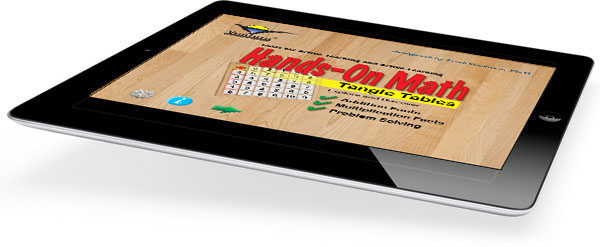\$2.99
 Tangle Tables Tangle Tables is a fun way to practice addition and multiplication skills. Using simple gestures students move number tiles on to an addition or multiplication table. Possible Topics: Addition Facts Multiplication Facts Factoring Problem Solving Relationship between Addition and Subtraction Relationship between Multiplication and Division Mathematical Reasoning Number Patterns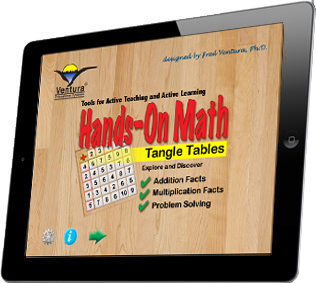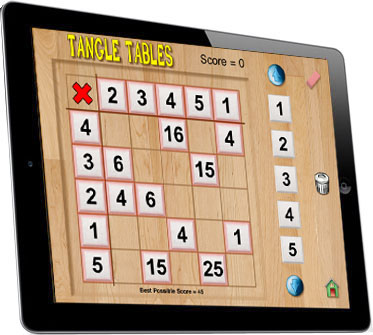Tangle Tables Functions By dragging an number tile the student can select a value and slide it into the table. Depending on the level setting the student may receive instantaneous feedback regarding the correctness of the tile placement. Select Levels by Tapping the Settings Icon Choose Addition or Multiplication Determine Range of Number Optional Sounds and Speech Functions Explore and Document Activities at a variety of skill levels are also provided. Students are encouraged to document their discoveries in a journal. A comprehensive teacher's guide provides lesson ideas at a variety of levels. Perfect for Small Group instruction Environmentally Friendly Learning - (no need to photocopy activity pages for each student) A Student-Centered Approach to Learning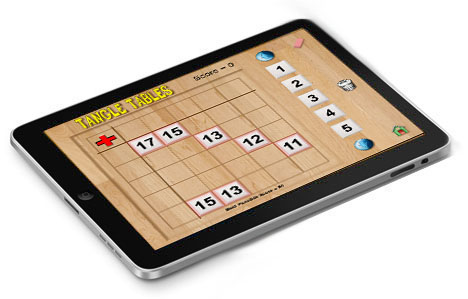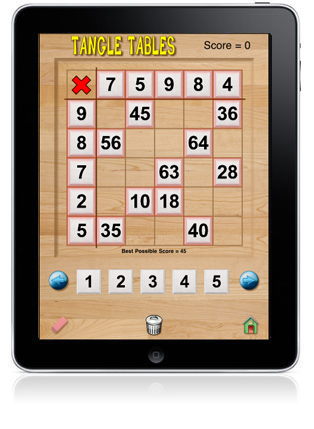Hands-On Math: Tangle Tables is now available at the Apple iTunes Store.